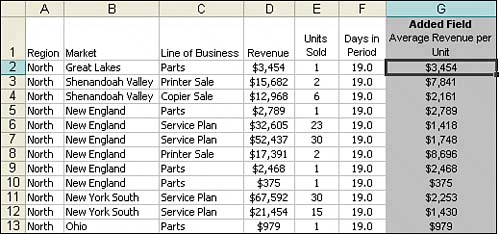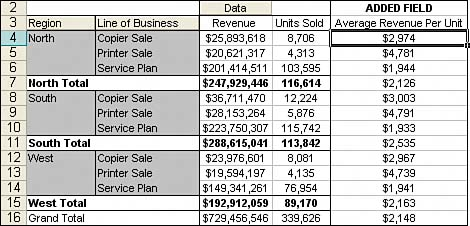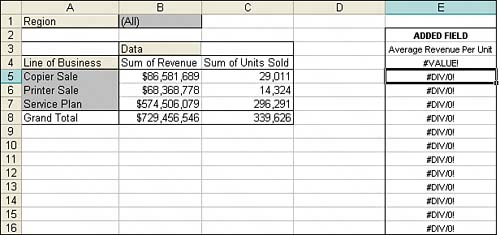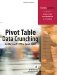# Introducing Calculated Fields and Calculated Items

When analyzing data with pivot tables, you will often find the need to expand your analysis to include data based on calculations that are not in your original dataset. Excel provides a way to perform calculations within your pivot table through calculated fields and calculated items.

A calculated field is a data field you create by executing a calculation against existing fields in the pivot table. Think of a calculated field as adding a virtual column to your dataset. This column takes up no space in your source data, contains the data you define with a formula, and interacts with your pivot data as a fieldjust like all the other fields in your pivot table.

A calculated item is a data item you create by executing a calculation against existing items within a data field. Think of a calculated item as adding a virtual row of data to your dataset. This virtual row takes up no space in your source data and contains summarized values based on calculations performed on other rows in the same field. Calculated items interact with your pivot data as a data item, just like all the other items in your pivot table.

With calculated fields and calculated items, you can insert a formula into your pivot table in order to create your own custom field or data item. Your newly created data becomes a part of your pivot table, interacting with other pivot data, recalculating when you refresh and supplying you with a calculated metric that does not exist in your source data.

The example in Figure 6.1 demonstrates how a basic calculated field can add another perspective to your data.

##### Figure 6.1. Average Revenue per Unit is a calculated field that adds another perspective to your data analysis.Your pivot table shows revenue and number of units sold by region and line of business. A calculated field that shows you average revenue per unit enhances this analysis and adds another dimension to your data.

Now you may look at Figure 6.1 and ask yourself, "Why go through all the trouble of creating calculated fields or calculated items? Why not just use formulas in surrounding cells or even add your calculation directly into the source table in order to get the information you need?"

To answer these questions, look at the different methods you could use to create the calculated field in Figure 6.1.

#### Method 1: Manually Add the Calculated Field to Your Data Source

You can manually add a calculated field to your data source, as shown in Figure 6.2, allowing the pivot table to pick the field up as a regular data field.

##### Figure 6.2. Precalculating calculated fields in your data source is both cumbersome and impractical.On the surface, it looks like a simple option, but this method of precalculating metrics and incorporating them into your data source is impractical on several levels.

Besides the fact that you increase the chance for errors by calculating and managing data with formulas, you limit your flexibility when requirements change.

If the definitions of your calculated fields change, you will have to go back to the data source, recalculate the metric for each row, and refresh your pivot table. If you have to add a metric, you will have go back to the data source, add a new calculated field, and then change the range of your pivot table to capture the new field.

#### Method 2: Use a Formula Outside of Your Pivot Table to Create the Calculated Field

You can add a calculated field by performing the calculation in an external cell with a formula. In the example in Figure 6.3, each cell in the Average Revenue per Unit column is made up of a formula that references the pivot table.

##### Figure 6.3. Typing a formula next to your pivot table will essentially give you a calculated field that refreshes when your pivot table is refreshed.Although this method will give you a calculated field that updates when your pivot table is refreshed, as you can see in Figure 6.4, any changes in the structure of your pivot table have the potential of rendering your formula useless.

##### Figure 6.4. Moving the Region field to the page area changes the structure of your pivot table, exposing the weakness of makeshift calculated fields that use external formulas.#### Method 3: Insert a Calculated Field Directly into Your Pivot Table

Inserting the calculated field directly into your pivot table is the best option. This eliminates the need to manage formulas, provides for scalability when your data source grows or changes, and allows for flexibility in the event that your metric definitions change.

Another huge advantage of this method is that you can alter your pivot table's structure and even measure different data fields against your calculated field without worrying about errors in your formulas or losing cell references.

The pivot table report shown in Figure 6.5 is the same pivot table you see in Figure 6.1, except it has been restructured so that you get the average revenue per unit by market.

##### Figure 6.5. Your calculated field remains viable even when your pivot table's structure changes to measure average revenue per unit for every market.The bottom line is that there are significant benefits to integrating your custom calculations into your pivot table. These benefits include the following:

• The elimination of potential formula and cell reference errors

• The ability to auto-recalculate when your pivot table is changed or refreshed

• The flexibility to change calculations easily when your metric definitions change

• The ability to manage and maintain your calculations effectivelyPivot Table Data Crunching for Microsoft Office Excel 2007
ISBN: 0789736012
EAN: 2147483647
Year: 2003
Pages: 140

Similar book on Amazon